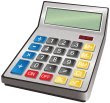# What is the Rule of 72?May 31, 2012: If you are planning to double your money, then The Rule of 72 is a quick way to determine how long it will take do so at a given interest rate.

How to calculate?
You divide the number 72 by the annual interest rate expected on your investment. The answer is the no. of years you will need to double your money at your specified interest rate compounded annually.

72 / Interest Rate = Years to Double

Examples?
Let's say you have an investing instrument that promises to pay you 8% APY. Then the Rule of 72 says that it would take 72/8 = 9 years to double your money at 8% APY.

Any other use of The Rule of 72?
In case you want to find out what APY (interest rate) it'd take to double your money in say 6 years, The Rule of 72 can still help you.

72 / Years to Double = Interest Rate

In this case divide 72 by the number of years you are willing to invest, i.e. 72/6 = 12. That means you would need an investing vehicle that will pay 12 percent interest compounded annually to double your money in 6 years.

To achieve FIRE we need to consistently double our money. Easier said than done :).

Image Source(s): iStockPhoto